# Regular scheme

(diff) ← Older revision | Latest revision (diff) | Newer revision → (diff)
A scheme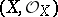such that at every point the local ring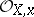is regular (cf. Regular ring (in commutative algebra)). For schemes of finite type over an algebraically closed field, regularity is equivalent to the sheaf of differentials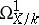being locally free. Regular local rings are factorial (cf. Factorial ring), and so any closed reduced irreducible subscheme of codimension 1 in a regular schemeis given locally by one equation (see ). An important problem is the construction of a regular schemewith a given fieldof rational functions and equipped with a proper morphism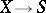onto some base scheme. The solution is known in the case whenis the spectrum of a field of characteristic 0 (see ), and for schemes of low dimension in the case of a prime characteristic and also in the case whenis the spectrum of a Dedekind domain with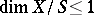(see ).